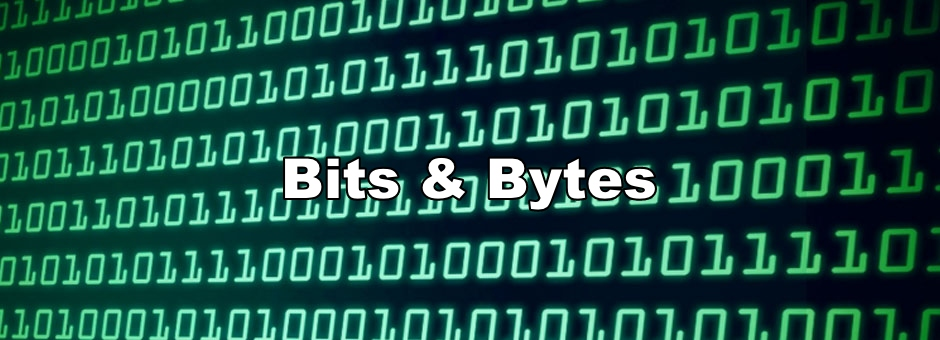Quick announcements

And… we’re back!

Sorry for the delay in newsletters. I’ve gotten feedback from folks that these newsletters are too often, so I’ve decided to turn this into a weekly newsletter.

If you’re new to the newsletter, as always, I post all of the previous newsletters on the Discord. Feel free to hop in and say hi :).Anyways, let’s go over the bitwise exercises from the previous email!

Here’s the link if you need a refresher or want to give them a shot now :).

## Bitwise Operators Exercises Debriefs

Write a method called zebra that returns an int with every other bit set to 1.

So for this problem, we want an integer of the form:

10101010 10101010 10101010 10101010

Now we can convert that to base 10 to get 2863311530. We could write a method that simply returns 2863311530, but that would defeat the purpose of this exercise!

One way we could approach this is by starting with 0 and iteratively change the bits 1 at a time. We can make use of the or operator, |, to flip bits and left shift operator, <<, to continuously shift bits.

To get a better picture of how this would work, let’s further break this down with what we expect on each iteration:

Iteration 0: 00000000 00000000 00000000 00000000

Iteration 1: 00000000 00000000 00000000 00000010

Iteration 2: 00000000 00000000 00000000 00001010

Iteration 3: 00000000 00000000 00000000 00101010

And so on and so forth until we get our final result.

On the first iteration, we’ll go ahead and incorporate the first 1 into the 0s. Let’s make use of the bit shift operator along with the number 1 to make sure the 1 gets put in the right spot:

00000000 00000000 00000000 00000001 << 1

Evaluates to:

00000000 00000000 00000000 00000010

Awesome, so we have the 1 in the right spot now! Let’s incorporate it with our resulting number:

00000000 00000000 00000000 00000000

00000000 00000000 00000000 00000010 |

Evaluates to:

00000000 00000000 00000000 00000010

So this matches up with the result from Iteration 1. In the next iteration, we need to shift bits over to the left to make room for the new 1. Again, we can make use of left shift again to push over the bits two spaces to the left:

00000000 00000000 00000000 00000010 << 2

Evaluates to:

00000000 00000000 00000000 00001000

Now we have space to add in another flipped bit again! We can do this until we’ve run this 16 times (since half of the 32 bits need to be flipped to be 1). Let’s go ahead and express this in Ruby code (don’t worry if you don’t know Ruby, it’s readable!):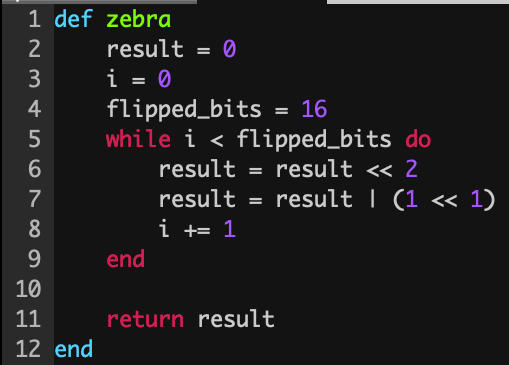And now we can check the result by firing up this into Ruby’s Read Evaluate Print Loop:

2.2.3 :002 > require './zebra.rb'

=> true

2.2.3 :003 > zebra

=> 2863311530

2.2.3 :004 > zebra.to_s(2)

=> "10101010101010101010101010101010"

There we go! It’s not so scary after all is it?

Write a method that takes in an integer x and another int, n, and flips the nth bit. That is, if the nth bit in the binary representation of integer x is 0, set it to 1, otherwise set it to 0.

So in other words, if we have an integer:

00000000 00000000 00001000 00000000

And n = 12, we’d flip the 1 to a 0:

00000000 00000000 00000000 00000000

Whenever we see setting a certain bit, this is a hint that the left bitwise operator will be an important player. This question is a little tricky if you’re not familiar with using all the bitwise operators.

We’re going to be making use of the XOR operation (denoted with a ^). Recall the definition of an XOR operator:

Bitwise XOR (^)

"Scans through the bits. If exactly one bit is set to 1, returns 1 for that bit, otherwise 0"

That’s exactly what we want!

The high level approach here is that we’ll shift a 1 to the nth bit. We’ll then make use of the XOR operation to XOR the number in question along with 1 << n. This 1 << n value is typically referred to as a bitwise mask. We typically make use of masks to filter out values that we’re not interested in.

Let’s demonstrate how this would work for the example we had above. Assume that we’re passed in 00000000 00000000 00001000 00000000 for our number and n = 12:

1 << 12 evals to:

00000000 00000000 00001000 00000000

Now we XOR the mask with the number passed in:

00000000 00000000 00001000 00000000

00000000 00000000 00001000 00000000 ^

Evals to:

00000000 00000000 00000000 00000000

Perfect! But what if n = 11?

1 << 11

Evals to:

00000000 00000000 00000100 00000000

XOR:

00000000 00000000 00001000 00000000

00000000 00000000 00000100 00000000 ^

Evals to:

00000000 00000000 00001100 00000000

Which is exactly what we wanted! Expressed in code: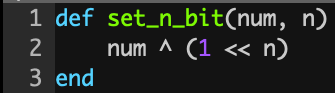And in the REPL:

2.2.3 :003 > set_n_bit(1 << 12, 12).to_s(2)

=> "0"

2.2.3 :004 > set_n_bit(1 << 12, 11).to_s(2)

=> "1100000000000"

Excellent! The last question is actually an interview question that’s asked by companies like Microsoft and Salesforce occasionally, so keep your eyes peeled.

A palindrome is defined as a sequence that reads the same backwards as forward. Write a function that takes in an integer and returns a boolean which denotes whether or not its binary representation is a palindrome. True if it’s a palindrome, false otherwise.

Besides reversing the element in question to see if it’s a palindrome and making sure the two values are equal, another way to tell if something is a palindrome is by keeping two pointers, one on the left hand side, and another on the right hand side. On each iteration, we would check if the values are equal until we cross over the middle of the string. Here’s an example of a function checking whether or not a palindrome is a string: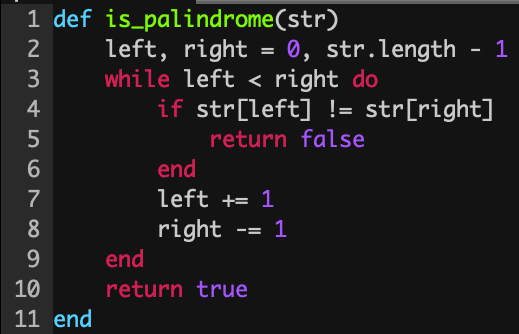We can apply the same approach to solving whether or not a number is a bitwise palindrome!

We’ll accomplish this by keeping track of two bitwise masks, one for the left side and one for the right side. First, we initialize the left hand side mask to 1 << 31 and the right hand side to 1. This gives us the two values:

10000000 00000000 00000000 00000000 = 1 << 31

00000000 00000000 00000000 00000001 = 1

Now we can use these masks in conjunction with the bitwise and operator, &, to extract the value at a certain bit position. Recall the definition of &:

Bitwise And

"Scans through the bits. If both bits are set to 1, returns 1 for that bit otherwise 0"

Now we can compare the values at these positions by using the masks. Let’s say we’re questioning whether or not the integer returned by the zebra method we wrote is a palindrome. The extraction process with the bitwise mask would look like as follows:

10101010101010101010101010101010

10000000000000000000000000000000 &

Evaluates to 10000000000000000000000000000000.

10101010101010101010101010101010

00000000000000000000000000000001 &

Evaluates to 0.

Note that we need to do some bitwise translation to massage the bits in a way where we can compare them. We can’t directly compare 10000000000000000000000000000000 and 0. We’ll need to make use of bit shift left or right to get them to be in a spot where we can compare them.

We can shift the left hand mask to the right so we can do the comparison:

10000000000000000000000000000000 >> 31

Evaluates to 1.

Now we can compare the values. 0 != 1, so we can return false early.

Let’s take an actual bitwise palindrome so we can get a better sense of how the algorithm would work. Let’s see how:

11000000 10000000 00000001 00000011

would fair.

11000000100000000000000100000011

10000000000000000000000000000000 &

Evals to:

10000000000000000000000000000000

11000000100000000000000100000011

00000000000000000000000000000001 &

Evals to:

00000000000000000000000000000001

We can’t compare the values quite yet. We need to do some more shifting so we can compare the values properly.

We can shift the left hand mask to the right so we can do the comparison:

10000000000000000000000000000000 >> 31

Evals to:

00000000000000000000000000000001

So we can go ahead and shift the left hand mask rightward one and the right hand mask leftward via bitwise operators. We see that 1 == 1, so we proceed to the next iteration by shifting both the left and right masks.

On the second iteration, we do the same two operations:

Left side:

11000000100000000000000100000011

01000000000000000000000000000000 &

01000000000000000000000000000000

Right side:

11000000100000000000000100000011

00000000000000000000000000000010 &

00000000000000000000000000000010

Now we need to shift the left side over a number of bits so we can compare the two values correctly. Notice that the number of bits that we need to shift over to the right shrunk from 31 down to 29 since the left mask shifted to the right by 1 and the right mask shifted to the left by 1.

The termination condition is almost the same here as well. As soon as the difference between the left mask and right mask is negative, we know that there are no more bits to compare.

Let’s replace some code from our original is_palindrome definition to get this to work: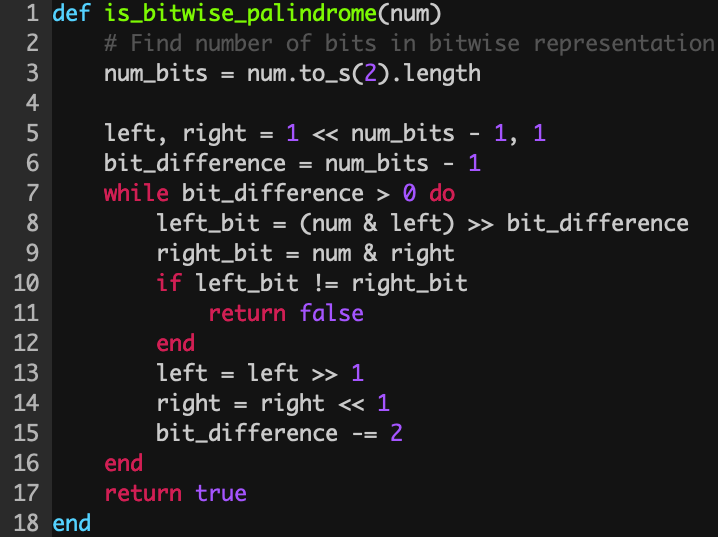And testing the solution:

2.2.3 :001 > require './palindrome.rb'
=> true
2.2.3 :002 > is_bitwise_palindrome("10000000100000000000000100000001".to_i(2))
=> true
2.2.3 :003 > is_bitwise_palindrome("100".to_i(2))
=> false

Wrapping up

Manipulating bits can be daunting at first, but hopefully going over these exercises helped solidify your understanding with bitwise operators. In next week’s edition of Beyond the Bootcamp, we’ll finally get to building out our very own BitSet!

As always, if there are any questions, feel free to reach out on the Discord or privately as well by responding to this email :).

Have a good week!

Justin

 Copyright © 2021 Gestone, All rights reserved. Want to change how you receive these emails? You can update your preferences or unsubscribe from this list.